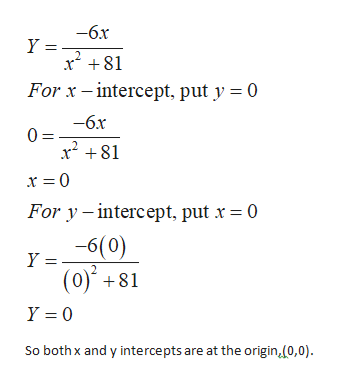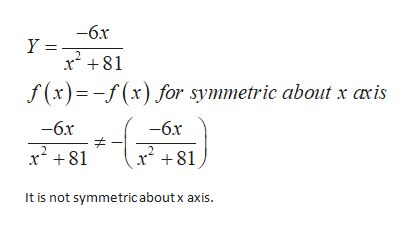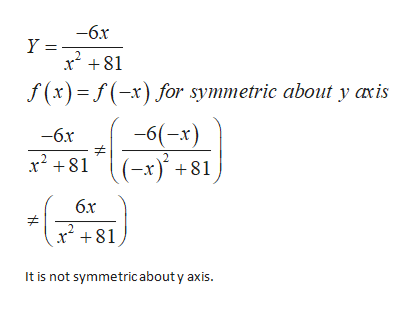For the given equation, list the intercepts and test for symmetry.  Y= -6x/x^2+81

Question

For the given equation, list the intercepts and test for symmetry.

Y= -6x/x^2+81

Step 1

Refer to the question,

To find the x and y intercepts put y=0 for x intercept and x=0 for y intercept.

Then,help_outlineImage Transcriptionclose-6x Y = r81 For x -intercept, put y 0 -6x 0= x281 For y -intercept, put x 0 y=6(0) (0)81 Y 0 So bothx and y intercepts are at the origin,(0,0) fullscreen
Step 2

Now to check for symmetry, a function is symmetric with respect to the x axis if f(a)=-f(a).

That is,help_outlineImage Transcriptionclose-6x Y =- 81 f(x)(x) for symmetric about x axis -6x -6x x81 x81 It is not symmetric about x axis fullscreen
Step 3

Then check symmetry about y axis, a function is symme...help_outlineImage Transcriptionclose-6x Y =- r81 f (x) x) for symmetric about y axis -6(-x) (-x) +81 -6x x81 6x x281 It is not symmetric about y axis. fullscreen

Want to see the full answer?

See Solution

Want to see this answer and more?

Our solutions are written by experts, many with advanced degrees, and available 24/7

See Solution
Tagged in

Algebra